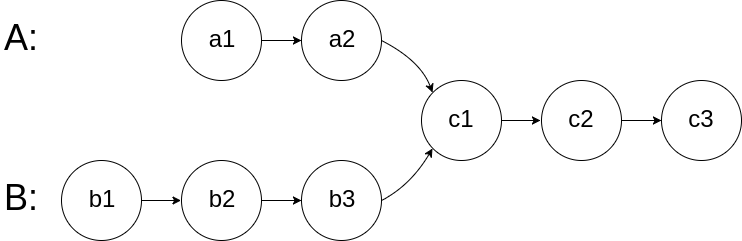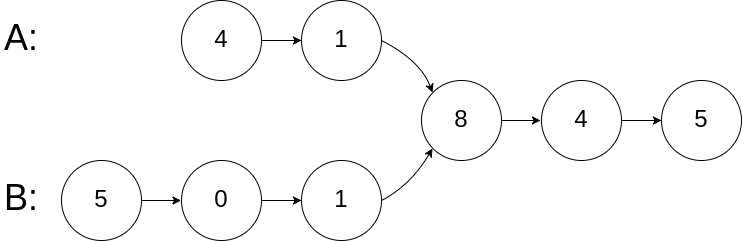160. Intersection of Two Linked Lists

Write a program to find the node at which the intersection of two singly linked lists begins.

For example, the following two linked lists:begin to intersect at node c1.

Example 1:```
Input: intersectVal = 8, listA = [4,1,8,4,5], listB = [5,0,1,8,4,5], skipA = 2, skipB = 3
Output: Reference of the node with value = 8
Input Explanation: The intersected node's value is 8 (note that this must not be 0 if the two lists intersect). From the head of A, it reads as [4,1,8,4,5]. From the head of B, it reads as [5,0,1,8,4,5]. There are 2 nodes before the intersected node in A; There are 3 nodes before the intersected node in B.

java：

``````/**

* public class ListNode {
*     int val;
*     ListNode next;
*     ListNode(int x) {
*         val = x;
*         next = null;
*     }
* }
*/
public class Solution {

while (lenA > lenB) {
lenA--;
}

while (lenB > lenA) {
lenB--;
}

}

}

int res = 0;
res++;